# Quiz 1: Introduction to Communication Systems

Trade & Technology

(a) Depending on the range of frequencies, the frequencies are classified into different frequency bands. The frequencies ranging from 3MHz - 30MHz are called as high frequencies (HF). High frequencies are used for standard time and frequency broadcasts. The frequency 10MHz lie in the range 3MHz - 30MHz. Therefore, the frequency 10MHz comes under the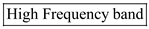. (b) The frequencies ranging from 300MHz - 3GHz are called as Ultra high frequencies (UHF). Ultra high frequencies used for microwave ovens, cellular telephones. The frequency 2.45 GHz lie in the range 300MHz - 3GHz. Therefore, the frequency 2.45 GHz comes under the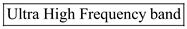. (c) The frequencies ranging from 30kHz - 300kHz are called as low frequencies (LF). Low frequencies are used LORAN navigation system for ships and aircraft. The frequency 100 kHz lie in the range 30kHz - 300kHz. Therefore, the frequency 100 kHz comes under the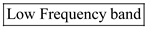. (d) The frequencies ranging from 3GHz - 30GHz are called as super high frequencies (SHF). Super high frequencies are used satellite television. The frequency 4 GHz lie in the range 3GHz - 30GHz. Therefore, the frequency 4 GHz comes under the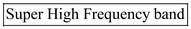. (e) The frequencies ranging from 300MHz - 3GHz are called as Ultra high frequencies (UHF). Ultra high frequencies used for microwave ovens, cellular telephones. The frequency 880 GHz lie in the range 300MHz - 3GHz. Therefore, the frequency 880 GHz comes under the.

(a) The transmitted information is of lowest frequency. Here, the voice frequency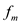is 400 Hz. The carrier frequency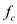is 1020 kHz. Therefore, the upper side frequency is,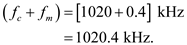And, the lower side band frequency is,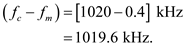Out of these two frequencies, the lowest frequency is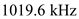at which the information gets transmitted. Since, the information frequency is less than the carrier frequency. Therefore, the information frequency is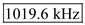. (b) The frequency of the carrier signal is the operating frequency of AM radio station. Therefore, the carrier frequency is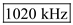. (c) Calculate the base band frequency.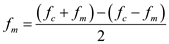Substitute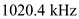for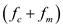, andfor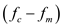.Therefore, the base band frequency is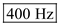. (d) As the carrier is modulated by a base band frequency, the modulating signal is composed of two different frequencies. Therefore, the upper side frequency is,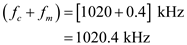And, the lower side band frequency is,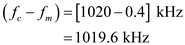Therefore, the modulating frequencies are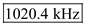and.

Modulation is a process in which the baseband signal (information signal) is superimposed on a high frequency carrier signal. The baseband signal is stored in the carrier signal. Modulation is used in telecommunication to transmit data via electrical signal. Base band signal can be added to carrier signal by varying its amplitude, frequency, and phase. Consider the sine carrier wave.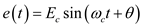The parameters of a sine wave carrier that can be modulated are the amplitude of the carrier signal (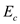), phase of the carrier signal (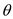), and the frequency of the carrier signal (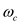). Depending on the variation of the parameter of the carrier signal, modulation is classified into three types: (1) Amplitude Modulation (2) Frequency modulation (3) Phase modulation If the amplitude of the carrier () changes according to the amplitude of the base band signal then it is called as Amplitude Modulation. If the frequency of the carrier signal () changes according to the amplitude of the base band signal then it is called as Frequency Modulation. If the phase of the carrier signal () changes according to the amplitude of the base band signal then it is called as Phase Modulation.